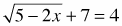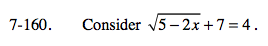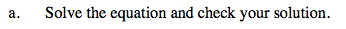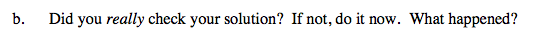Home > A2C > Chapter 7 > Lesson 7.3.1 > Problem7-160

7-160.
1. Consider. Homework Help ✎
.

1. Solve the equation and check your solution.

2. Did you really check your solution? If not, do it now. What happened?Subtract 7 from both sides and square both sides to remove the square root.$-\sqrt{A}\text{ can equal}-3, \text{ but can}\sqrt{A} = -3?$